### IMO Shortlist 2002 problem G3

Kvaliteta:
Avg: 0,0
Težina:
Avg: 7,0
The circle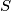$S$ has centre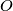$O$, and$BC$ is a diameter of$S$. Let$A$ be a point of$S$ such that$\angle AOB<120{{}^\circ}$. Let$D$ be the midpoint of the arc$AB$ which does not contain$C$. The line through$O$ parallel to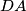$DA$ meets the line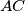$AC$ at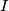$I$. The perpendicular bisector of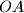$OA$ meets$S$ at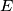$E$ and at$F$. Prove that$I$ is the incentre of the triangle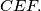$CEF.$
Izvor: Međunarodna matematička olimpijada, shortlist 2002